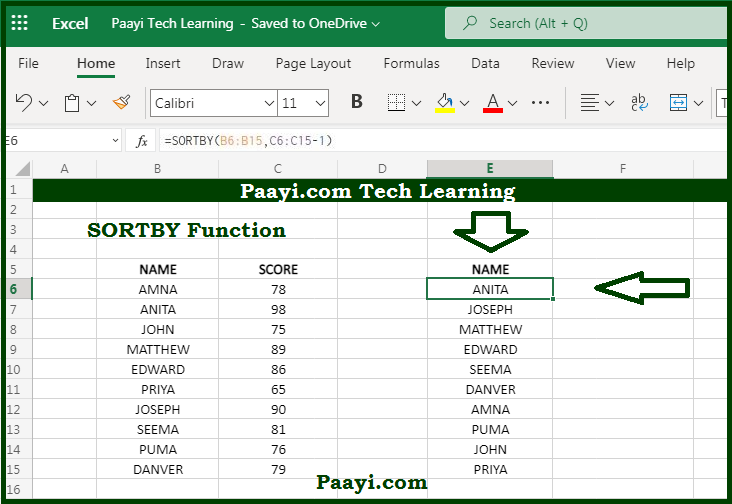# Learn How to Use Microsoft Excel SORTBY Function

Written by | 0 Comments | 612 Views

In this article, you will learn how to use the Microsoft Excel SORTBY function and its prime function in Microsoft Excel. You will also get to know the Microsoft Excel SORTBY function return value and syntax with the help of some examples.

Microsoft Excel SORTBY Function

The main function of Microsoft Excel SORTBY function is to SORTBY range or an array. That implies with the help of the SORTBY function; you can able to SORTBY the content or range or an array. You can SORTBY the values by one or more columns, and the SORTBY function returns the dynamic arrays of results.

Return Value of SORTBY Function

The return value will be sorted array.

Syntax of SORTBY Function

=SORTBY(array, by-array, [sort-order], [array/order])

Where the arguments:

array: This is the range or array you want to SORT.

by-array: This is the range or array you want to sort by.

sort-order: 1 = Ascending, -1 = Descending, default is ascending order (optional).

array/order: This is the additional array and sort order pairs (optional).

## How to Use Microsoft Excel SORTBY Function?So, you know now that with the help of the Microsoft Excel SORTBY function, you can able to sort the contents of the array or range according to the values from another range or array. The range or array used to sort may not appear in the source data.